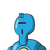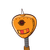# If 10 men can build a wall in 5 days then in how many days 5 men can build he same wall

If 10 men can build a wall in 5 days then in how many days 5 men can build he same wall

### 2 thoughts on “If 10 men can build a wall in 5 days then in how many days 5 men can build he same wall”

1.### Given:

• If 10 men can build a wall in 5 days

### ToFind:

• In how many days will 5 men build the same wall

### Solution:

Now,

• Let the number of day taken by 5 men to build the wall be x days

Table :

$$\begin{gathered}\begin{gathered}\begin{gathered} \tiny\boxed{\begin{array}{ c |c} \frak{ \pmb{men}}& \rm{ \pmb{days}}\\ \dfrac{\qquad\qquad}{ \sf 10}&\dfrac{\qquad\qquad}{ \sf 5 \: days}& \\ \dfrac{\qquad\qquad}{ \sf 5}& \dfrac{\qquad\qquad}{ \sf x \: days} \end{array}}\end{gathered}& \\ \end{gathered}\end{gathered}$$

Here,

• As the men of decrease the no. of days to do the work will increase so they are in inverse proportion

When,

• Two quantities are in inverse proportion we direct multiply the means and extreme terms on both the side and transpose numericals to find the value of the variables .

$$\longrightarrow \tt \: 10 : 5 \propto 5 : x \\ \\ \\ \longrightarrow \tt \: 50 = 5x \: \: \: \: \: \: \: \: \: \: \\ \\ \\ \longrightarrow \tt \: x = \cancel \frac{50}{5} \: \: \: \: \: \: \: \: \: \: \\ \\ \\ \longrightarrow \tt { \boxed{ \frak{x = 10 \: days}}}$$

• Henceforth, 5 men can build the wall in 10 days

### Method2

We know,

• 10 men can build up the wall in 5 days.

So,

• One man can build the wall in

$$\longrightarrow \tt \: 10 \times 5 \: days \\ \\ \\ \longrightarrow \: { \pink{ \boxed{ \tt{ 50 days}}}}\: \: \: \:$$

Now,

• 5 men can build the wall in

$$\longrightarrow \tt \: 50 \div 5 \: days \\ \\ \\ \longrightarrow { \blue{ \boxed{ \tt{10days}} \star}} \: \: \:$$

### Hence:

• 5 men can build the wall in 10 days

### Moretoknow:

• When two ratios are in direct proportion

$${ \orange{ \star{ \boxed{ \tt \: {products \: of \: means \: = products \: of \: extremes}}}}}$$

Here,

• You multiply the means of both sides and extremes of both the sides and find the value of the variable term transposition the numericals
2.Correct Question :-

If 10 men can build a wall in 5 days. Then in how many days 5 men can build the same wal?

Given:

• 10 men can build a wall in 5 days.

To find:

• How many days 5 men can build he same wall?

Solution:

• Let’s consider days Required for 5 men to build the same wall be x.

10,5,5 & x are given to us.

What to do?

We need to find x.

• 10,5,5,x

Step-by-step explanation :-

Here, 10 men can build a wall in 5 days. We have to find out x(how many days 5 men can build the same wall).

10 : 5 = x : 5

→ 10/5 = x/5

→ 5x = 5 × 10

→ 5x = 50

→ x = 50/5

x = 10

∴ Hence, 5 men can build the same wall in 10 days.